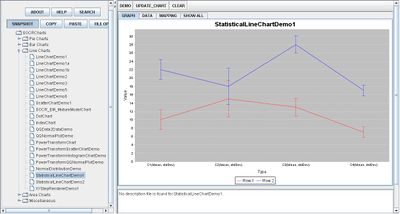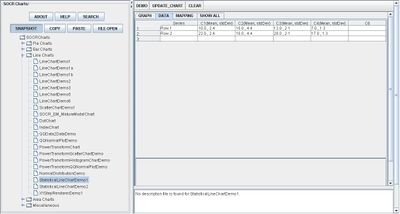# SOCR EduMaterials Activities StatisticalLineChart

(diff) ← Older revision | Latest revision (diff) | Newer revision → (diff)

## Summary

This activity describes the need, general methods and SOCR utilities for statstical line chart.

## Goals

The goals of this activity are to:

• gain a better understanding of the statistical line chart
• allow users to explore the statistical line chart with SOCR

## Background

Line Charts may also display statistical values in charts known as Statistical Line Charts. These charts do not have plotted values but instead they display the range mean and standard deviation of the independent variables.

For references, visit Line Charts and More Different Types of Line Charts.

## Description

Go to the SOCR Charts and select Line Charts from the items located on the left, then select the demonstration for Statistical Line Charts:The image above is a demonstration of a Statistical Line Chart. Notice that for each independent variable labeled, the mean and standard deviation is visually graphed. Observers are able to determine the mean and range of the specific variable of both events.

## Examples & Exercises

• Exercise 1: What are some differences and similarities between the Statistical Line Chart and the Normal Distribution?
• Exercise 2: If you are given the scores of a final, can conclusions be drawn when a statistical line chart is used to display the results?

## Data Type and Format

By clicking Data between the Graph and Mapping button, it allows users to input or vary the values of the data set. In this type of chart, the statistical values of each event is needed to create plots on the graph. The image below demonstrates this by listening the mean and standard deviation for the corresponding event:## Applications

One of the most persuasive elements when proposing data and literature to others is a well-designed chart presentation.

Suppose an experiment proposes one or more different variables, each with a range of values. By utilizing the Statistical Line Chart, graphing the mean and standard deviation for each variable may present the data set in a simple matter.

For example, students have five different brands of beverages. They want to compare the mean weight of the companies to determine if the stated weights on the cans are true or estimated. After all the data is collected, they calculate the mean and standard deviation for each beverage and graph them on a Statistical Line Chart. From this chart, observers are able to observe the statistical values of the different beverages as well as discover which brand offers the true mean weight of its beverage.